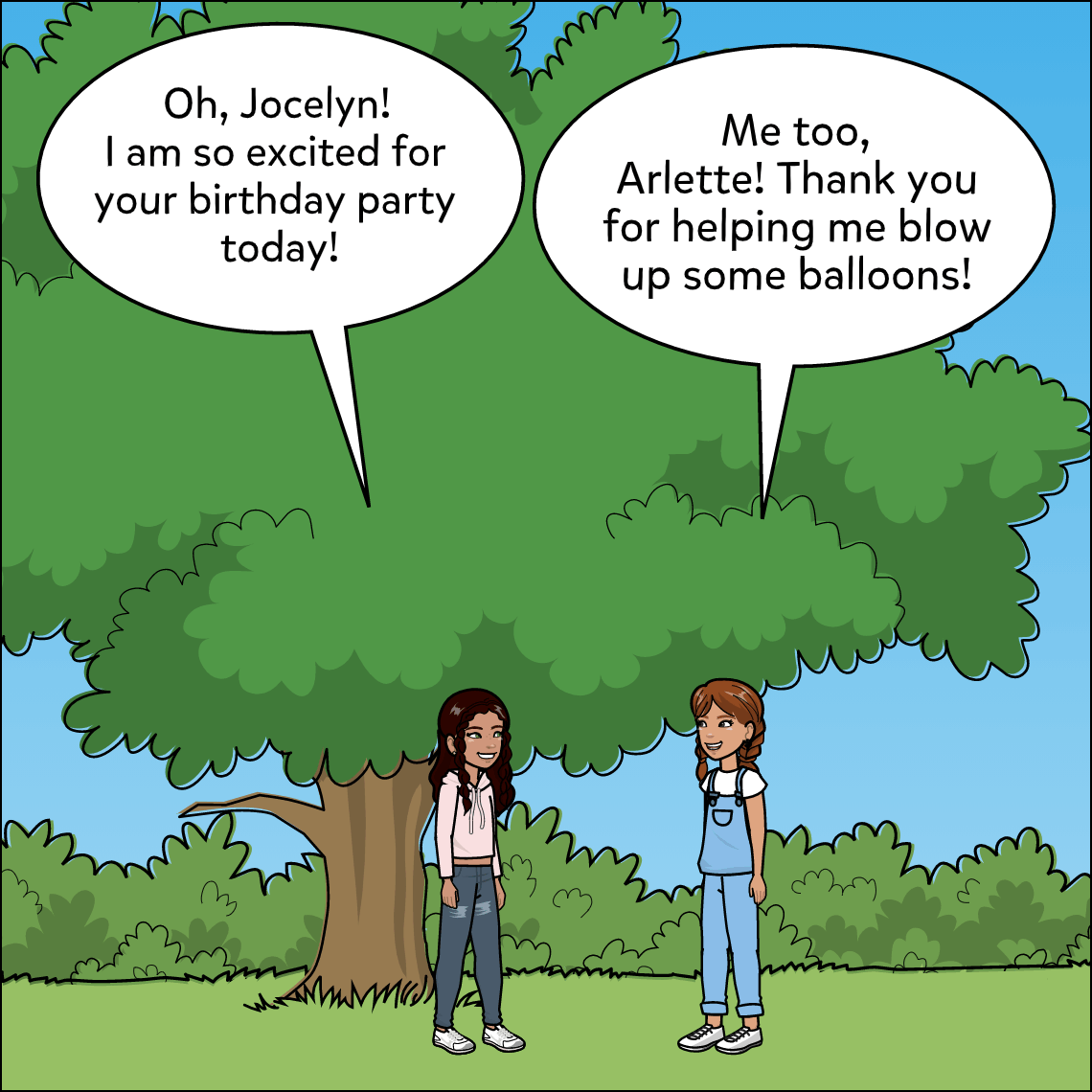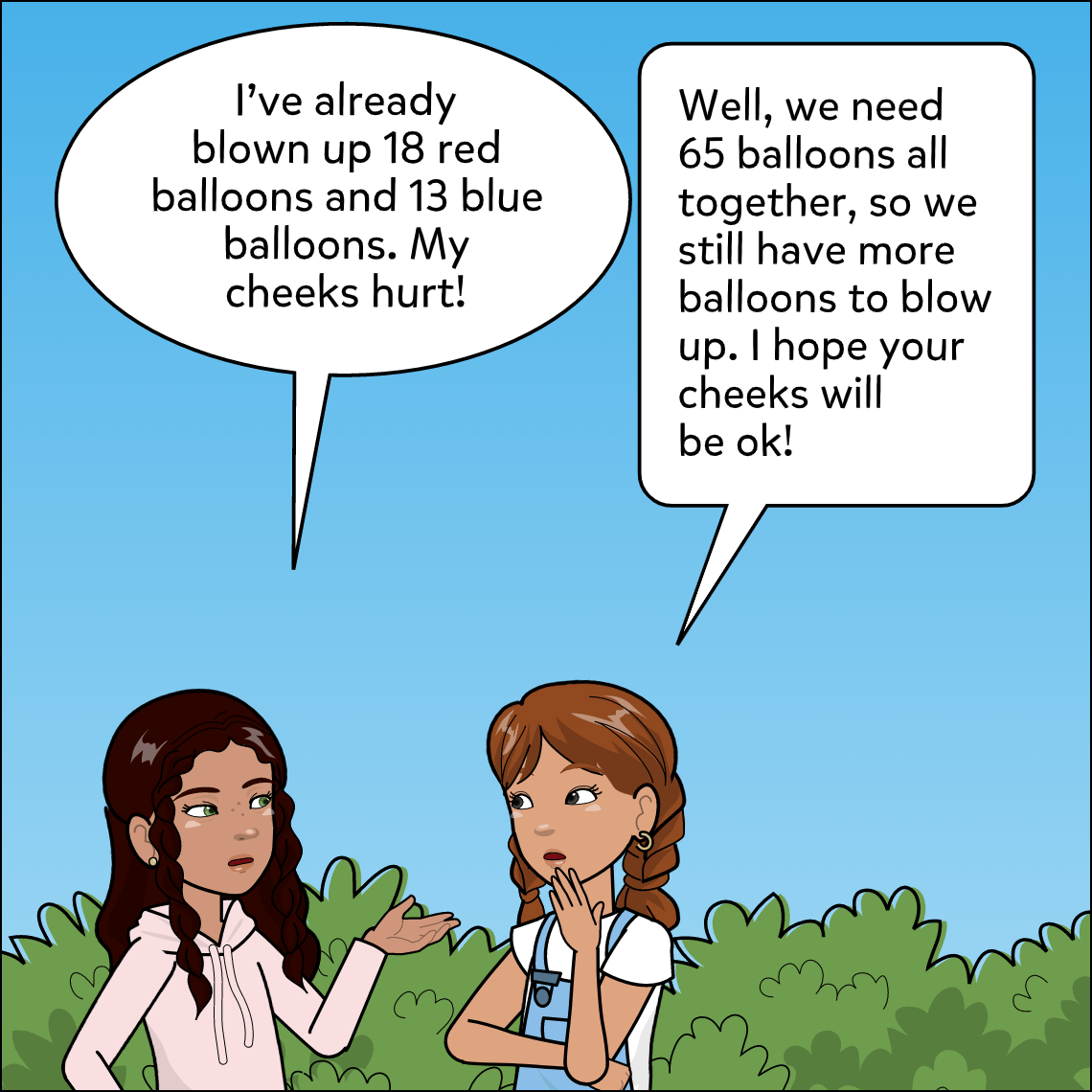Subject: Math

Lesson Length: 30 min.

Topic: Solving Word Problems With Subtraction

Objective: Evaluation, Narration

Standards: CCSS.MATH.CONTENT.3.OA.D.8

Standards:

Solve two-step word problems using the four operations.

Brief Description: Students will better understand addition and subtraction by solving and creating multi-step word problems.

Know Before You Start:

• Students should have an understanding of how addition and subtraction are used in daily life.
• Have a list of subtraction problems that students can pick from to create their comic.Hook:

• Read and discuss the sample comic.  Which operations will Arlette need to use to solve the problem? Are there any key words that helped?
• Solve the word problem as a class.

Activity:

• Assign students a subtraction problem and have them create a comic to demonstrate their understanding.
• As in the sample, the comic should depict a real life subtraction problem.
• The last panel should show the solution to their problem.

Closure: As a class or in small groups, have students share and discuss their word problem comics.

Differentiation:

• Provide more complex or simplistic subtraction problems as needed
• Allow students to use the speech-to-text feature as needed
• Have real-life examples ready for students to choose from

Resources: Example comic to print or display: Comic 1.